# Planimetrics - math problems

Study plane measurements, including angles, distances, and areas. In other words - measurement and calculation of shapes in the plane. Perimeter and area of plane shapes.

#### Number of problems found: 2259

• ABS trianglePoint S is the center of the square ABCD. The ABS triangle has a content of 9 cm2. What is the circumference of the square in cm?
• Wooden prism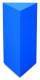Find the weight of a wooden regular triangular prism with a height equal to the perimeter of the base and a figure inscribed in a circle with a radius of 6, M cm, where M is the month of your birth. The density of oak is 680 kg/m3.
• Isosceles triangle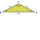Calculate the height of the isosceles triangle ABC with the base AB, AB = c = 10 cm and the arms a = b = 13 cm long.
• Two forces 3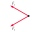Two forces of magnitude 8 Newtons and 15 Newtons respectively act at a point. If the resultant force is 17 Newtons, find the angle between the forces.
• Trapezoid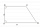The rectangular trapezoid ABCD with right angle at the vertex A has sides a, b, c, d. Calculate the circumference and the area of the trapezoid if given: a = 25cm, c = 10cm, d = 8cm
• Calculate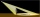Calculate the height of a tree that casts a shadow 22 m long, if you know that at the same time a pillar 2 m high casts a shadow 3 meters long.
• Squares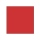From the square with the integer side, cut out the square with the integer side so that the residual area is 100. What is the longest possible side of the larger square?
• Rectangular lotWhat is the area of a rectangular lot measures 12 3/4 meter long and 8 5/6 wide?
• Find the 29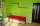Find the area of a room 9/7 m and 2/7 m.
• Two angles 2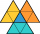Two angles of a triangle have the same measure and the third one is 45 degrees greater than the measure of each of the other two. Find the measure of the largest angle in the triangle.
• Sides in ratio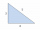The sides of the triangle are in a ratio of 2: 8: 5. Find the dimensions of the remaining sides if the longest side is 32 cm.
• Trapezoid and diagonals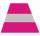Find the area of a trapezoid with bases a = 24 cm, c = 16 cm and diagonals u = 22 cm, v = 26 cm.
• The length 8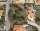The length of a rectangular field is x + 4 and its width is x + 2. what is the area of the field? Answer in polynomials expressions.
• Similarity of triangles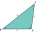If triangle ABC ~ to triangle XYZ, AC = 24, AB = 15, BC = 17, and XY = 9, what is the perimeter of triangle XYZ? Round all sides to 1 decimal place.
• The block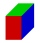The block has the dimensions of 5 cm, 10 cm, and 15 cm. Calculate the size of the wall diagonals of this block.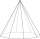You need to make a pad in the shape of a regular octagon with a side length of 4 cm. What is the minimum diameter of the circle-shaped semi-finished product from which we make the pad, and what will be the percentage of waste? (Round the results to 1 deci
• What is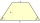What is the circumference of an isosceles trapezoid with a content of 106.75 cm 2 , the lengths of the sides are in the ratio 1: 3: 2: 1 and the bases are 6.1 cm apart?
• Show that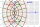Show that the mapping w = z +c/z, where z = x+iy, w = u+iv and c is a real number, maps the circle |z| = 1 in the z-plane into an ellipse in the (u, v) plane.
• Countertop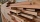Sam it is constructing he measures a rectangle area are on the countertop of 18 1/2 x 35 1/3” he will need to cut his area out in order to place his sink and the opening. What is the product of the two measurements round to the nearest whole?
• Height of the prism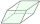The volume of the quadrilateral prism is 723.6 cm3. The base of this prism is a rhombus with a side 9 cm long and a corresponding height of 6.7 cm long. Find the height of the prism.

Do you have an exciting math question or word problem that you can't solve? Ask a question or post a math problem, and we can try to solve it.

We will send a solution to your e-mail address. Solved examples are also published here. Please enter the e-mail correctly and check whether you don't have a full mailbox.